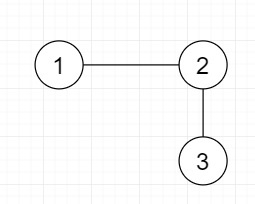# Minimum edges required to add to make Euler Circuit in Python

Suppose we have an undirected graph of b number of nodes and a number of edges; we have to find minimum edges needed to build Euler Circuit in this graph.

So, if the input is likethen the output will be 1.

To solve this, we will follow these steps −

• Define a function dfs() . This will take g, visit, odd_vert, degree, comp, v
• visit[v] := 1
• if degree[v] mod 2 is same as 1, then
• odd_vert[comp] := odd_vert[comp] + 1
• for u in range 0 to size of g[v], do
• if visit[u] is same as 0, then
• dfs(g, visit, odd_vert, degree, comp, u)
• From the main method do the following −
• g := a list of n+1 blank lists
• e := a new list
• := a new list
• degree := new list of size (n + 1) and fill with 0
• visit := new list of size (n + 1) and fill with 0
• odd_vert := new list of size (n + 1) and fill
• with 0
• for i in range 0 to m, do
• insert d[i] at the end of g[s[i]]
• insert s[i] at the end of g[d[i]]
• degree[s[i]] := degree[s[i]] + 1
• degree[d[i]] := degree[d[i]] + 1
• ans := 0, comp := 0
• for i in range 1 to n + 1, do
• if visit[i] is same as 0, then
• comp := comp + 1
• dfs(g, visit, odd_vert, degree, comp, i)
• if odd_vert[comp] is same as 0, then
• insert comp at the end of e
• otherwise,
• insert comp at the end of o
• if size of o is same as 0 and size of e is same as 1, then
• return 0
• if size of o is same as 0, then
• return size of e
• if size of e is not same as 0, then
• ans := ans + size of e
• for i in range 0 to size of o, do
• ans := ans + odd_vert[i] / 2 (take only integer part)
• return ans

## Example

Let us see the following implementation to get better understanding −

Live Demo

def dfs(g, visit, odd_vert, degree, comp, v):
visit[v] = 1
if (degree[v] % 2 == 1):
odd_vert[comp] += 1
for u in range(len(g[v])):
if (visit[u] == 0):
dfs(g, visit, odd_vert, degree, comp, u)
def solve(n, m, s, d):
g = [[] for i in range(n + 1)]
e = []
o = []
degree =  * (n + 1)
visit =  * (n + 1)
odd_vert =  * (n + 1)
for i in range(m):
g[s[i]].append(d[i])
g[d[i]].append(s[i])
degree[s[i]] += 1
degree[d[i]] += 1
ans = 0
comp = 0
for i in range(1, n + 1):
if (visit[i] == 0):
comp += 1
dfs(g, visit, odd_vert, degree, comp, i)
if (odd_vert[comp] == 0):
e.append(comp)
else:
o.append(comp)
if (len(o) == 0 and len(e) == 1):
return 0
if (len(o) == 0):
return len(e)
if (len(e) != 0):
ans += len(e)
for i in range(len(o)):
ans += odd_vert[i] // 2
return ans
b = 3
a = 2
source = [ 1, 2 ]
destination = [ 2, 3]
print(solve(b, a, source, destination))

## Input

b = 3 a = 2 source = [ 1, 2 ] destination = [ 2, 3]

## Output

1